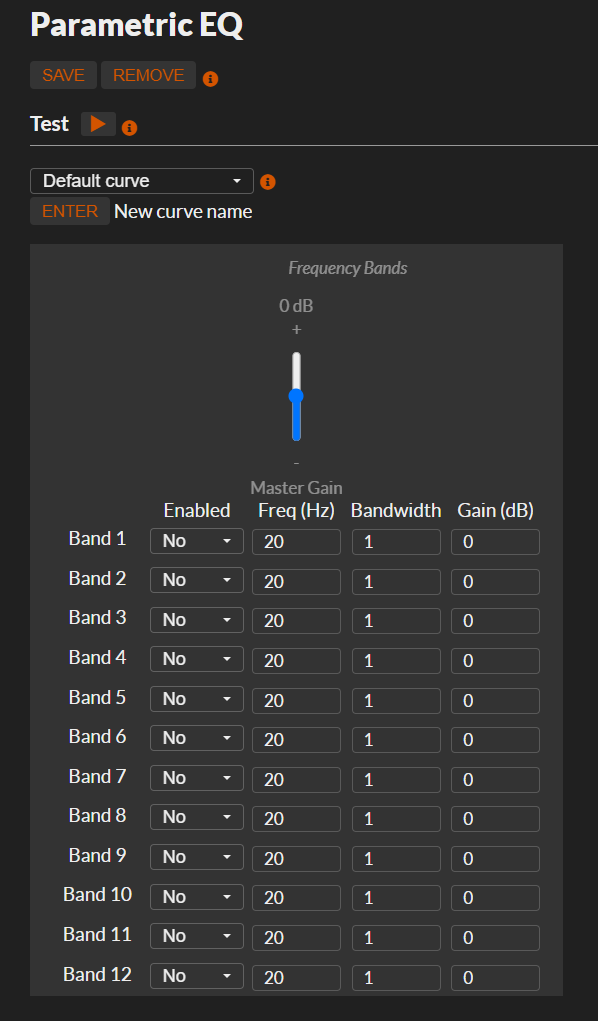# moOde with AutoEq headphone equalizing

2020-10-29: The new 12 band parametric Eq will be part of of the upcoming moOde 7.0.0 release

The AutoEq project provides headphone equalize settings. The equalizing requires at least a 5 band parametric equalizer and would prefer a 10 bands for optimal results. Standard moOde only provide a fourfold parametric equalizer.

The used parametric equalizer in moOde is the EqFa4p from the CAPs project.

Quoted from the caps website:

Four Mitra-Regalia peaking equaliser filters in series; a vector arithmetic re-implementation of Fons Adriaensens “Parametric1” equaliser[fafil] with minor differences.
Parallelisation of the serial filter organisation causes the output to lag by three samples.

With playback the output lag isn’t a concern, I have created a version of the equalizer with 3 x 4 parallel filters.

By lack of an official repo to fork from, I put the latest 0.9.26 source from the caps website im my own Github repo at bitkeeper/caps . The branch eqfa12p_12_band_parametric_eq contains a caps version with an EqFa120p.

Ok technical the name should EqFa3x4p and not 12p, but for now it is EqFa12p.

## Getting, build and installing the new caps library

```git clone --single-branch --branch features/eqfa12p_12_band_parametric_eq  https://github.com/bitkeeper/caps.git
cd caps
./configure.py
make
sudo make install```

## Let moOde use the EqFa12p

First to allow alsa to find the new filter we have to register the plugin by creating (requires sudo) a file called `/etc/alsa/conf.d/eqfa12p.conf` with the following content:

```pcm.eqfa12p {
type plug
slave.pcm "plug_eqfa12p"
}

pcm.plug_eqfa12p {
type ladspa
slave.pcm "plughw:2,0";
path "/usr/lib/ladspa";
plugins [ {
id 2611
label EqFA12p
input {
controls [1 14 1.471 6.0  1 1365 1.282 10.4  1 3212 0.442 5.6  1 4600 10.000 -9.5  1 11179 0.510 11.5  1 4572 0.110 3.1  1 6779 0.265 -4.6  1 8996 0.193 5.0  1 11627 0.175 -2.6  1 15559 0.276 2.1   0 20 1 0  0 20 1 0  -6.5]
}
} ]
}
```

The line that start with controls (all values should be on one single line) contains the setttings exactly the same as with the EqFa4p; per band enable/disable, frequency,bandwidth and gain. Add the end single which is the overall gain. In the example are the setting for my Sennheisser HD800S used.

Mostly with parametric eqs the Q is used instead of bandwidth parameter. The filter bandwidth factor could normally be calculatedby deviding the center frequency by the Q factor. Only it doesn’t seems to be correct with EqFa.

After reading the excellent article Parametric Equalization Theory: Relationship of BW, Q, fo & 3dB points, it was possible to calculate the frequencies for bandwidth of a certain Q. From this it seems that you have to devide the bandwith factor by 2 to get the correct EqFa bw parameter.

As example (generated with a piece of Python code):

```fc=14.00Hz    Q=0.34  bw=2.94 fl=4.3 fh=45.5 fd=41 bw_eqfa=1.47
fc=1365.00Hz Q=0.39  bw=2.56 fl=469.4 fh=3969.4 fd=3500 bw_eqfa=1.28
fc=3212.00Hz  Q=1.13    bw=0.88 fl=2091.1 fh=4933.6 fd=2842 bw_eqfa=0.44
fc=4600.00Hz Q=0.05 bw=20.00 fl=229.4 fh=92229.4 fd=92000 bw_eqfa=10.00
fc=11179.00Hz Q=0.98  bw=1.02 fl=6846.4 fh=18253.5 fd=11407 bw_eqfa=0.51
fc=4572.00Hz  Q=4.53  bw=0.22 fl=4095.1 fh=5104.4 fd=1009 bw_eqfa=0.11
fc=6779.00Hz  Q=1.89   bw=0.53 fl=5218.8 fh=8805.6 fd=3587 bw_eqfa=0.26
fc=8996.00Hz  Q=2.59  bw=0.39 fl=7425.4 fh=10898.8 fd=3473 bw_eqfa=0.19
fc=11627.00Hz  Q=2.86  bw=0.35 fl=9770.7 fh=13836.0 fd=4065 bw_eqfa=0.17
fc=15559.00Hz Q=1.81   bw=0.55 fl=11843.7 fh=20439.8 fd=8596 bw_eqfa=0.28```

With this knowledge is is easy to ‘translate’ the parametric equalizer settings provided by AutoEq. Make sure you setup the controls you your required settings.

Next we should prep mpd to use the EqFa12p instead of the EqFa4p.

As temporary solution you can just edit the mpd configuration file (requires sudo) in `/etc/mpd.conf`. Replace the device name as below.

```audio_output {
type "alsa"
name "ALSA parametric eq"
device "eqfa12p"
mixer_type "none"
dop "yes"
}

If moode is restarted (or the pi rebooted) it will overwrite this file. It isn't a final solution but good enough to play arround a little bit. Now we restart only mpd by:```
`sudo systemctl restart mpd`

If you run the command mpc you can inspect the current used output:

```pi@moode:~/caps \$ mpc outputs
Output 1 (ALSA default) is enabled
Output 2 (ALSA crossfeed) is disabled
Output 3 (ALSA parametric eq) is disabled
Output 4 (ALSA graphic eq) is disabled
Output 5 (ALSA polarity inversion) is disabled
Output 6 (ALSA bluetooth) is disabled
Output 7 (HTTP stream) is disabled
```

We nog have to select the third output with the command:

```pi@moodep4:~/caps \$ mpc enable only 3
Output 1 (ALSA default) is disabled
Output 2 (ALSA crossfeed) is disabled
Output 3 (ALSA parametric eq) is enabled
Output 4 (ALSA graphic eq) is disabled
Output 5 (ALSA polarity inversion) is disabled
Output 6 (ALSA bluetooth) is disabled
Output 7 (HTTP stream) is disabled
```

If we start the playback of a track, the audio stream is send to the EqFa12p.

## Validation

Now everything is in place, it is time for the validation. That is the most easy to see by measuring the bandwidth between 20Hz and 20kHz,

For the measuring I use:

• PC with:
• RightMark Audio Analyzer
• Asus Xonar D2X SPDIF Input
• moOde Pi
• Output device with SPDIF

With RMAA you can generate test signals as waveform for playback from the Pi. All due the Xonar D2X has nice specs for the analog in- and output, today we will stay digital and use the SPDIF input.

If we compare the bandwidth curve with the required transmission on AudioEq the form is pretty ok. Only the doesn’t match; the dip around 6 + kHz should be lower and around 10k should also be a little be lower. For a first try I’m quite satisfied.

## Integration into moOde

The 12 band parametric EQ will replace the current 4 band Eq in moOde version 7. No need to hassle with the scripts above.moOde 12 band parametric EQ